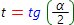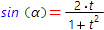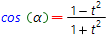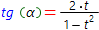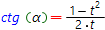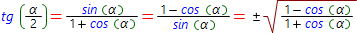# Trigonometric formulas

## 1. Identities of the trigonometric functions of same the argument: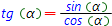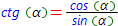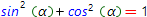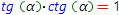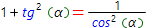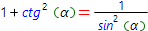## 2. Values of the trigonometric functions at specific points:

α° 0 30 45 60 90 180 270 360
sin(α) 0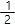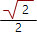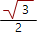1 0 -1 0
cos(α) 10 -1 0 1
tg(α) 0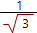1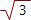0 0
ctg(α)10 0

## 3. Evenness or oddness: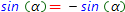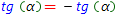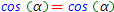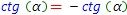## 4. The signs at quarters:

Trig. function Quater
I II III IV
sin(α) + +
cos(α) + +
tg(α) + +
ctg(α) + +

## 5. Reduction formulas:

β = 90 ± α 180 ± α 270 ± α 360 ± α
sin(β) cos(α) ∓sin(α) cos(α) ±sin(α)
cos(β) ∓sin(α) cos(α) ±sin(α) cos(α)
tg(β) ∓ctg(α) ±tg(α) ∓ctg(α) ±tg(α)
ctg(β) ∓tg(α) ±ctg(α) ∓tg(α) ±tgctg(α)

## 6. Solution of the simplest trigonometric equations:

sin(x) = a ⇔ x = (-1)n arcsin(a) + n π, n = 0, 1 ... ∈ Z
cos(x) = a ⇔ x = ± arccos(a) + 2 n π, n = 0, 1 ... ∈ Z
tg(x) = a ⇔ x = arctg(a) + n π, n = 0, 1 ... ∈ Z

## 7. Sum and subtraction formulas: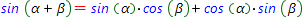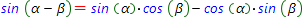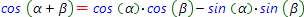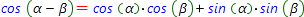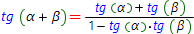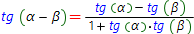## 8. Double-angle formulas: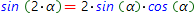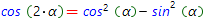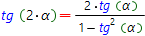## 8. Triple-angle formulas: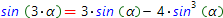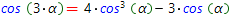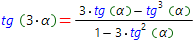## 9. Sum and difference transformation formulas:

### Sine transformations: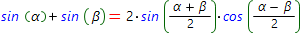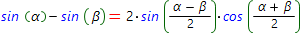### Cosine transformations: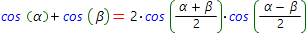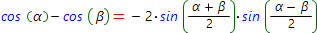### Tangent transformations: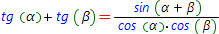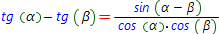### Cotangent transformations: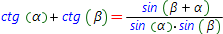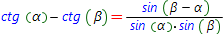### b) Transformation of the sum and difference of the different trigonometric functions with different angles: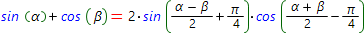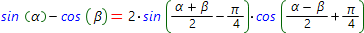### c) Specific formulas: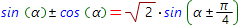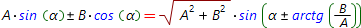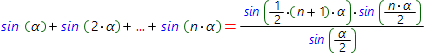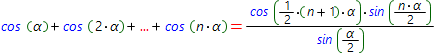## 10. Trigonometric functions product transformation formulas: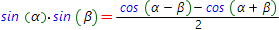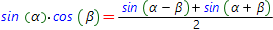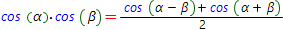## 11. Reduce trigonometric functions power formulas: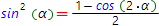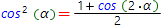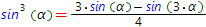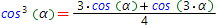## 11. Expression of the trigonometric functions by the tangent of the half angle: